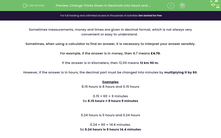# Change Times Given in Decimals into Hours and Minutes

In this worksheet, students use a calculator to convert times given in decimals to times expressed in hours and minutes.Key stage:  KS 3

Curriculum topic:   Number

Curriculum subtopic:   Use Standard Units of Measure

Difficulty level:#### Worksheet Overview

Sometimes measurements, money and times are given in decimal format, which is not always very convenient or easy to understand.

Sometimes, when using a calculator to find an answer, it is necessary to interpret your answer sensibly.

For example, if the answer is in money, then 4.7 means £4.70.

If the answer is in kilometers, then 12.09 means 12 km 90 m.

However, If the answer is in hours, the decimal part must be changed into minutes by multiplying it by 60.

Examples

8.15 hours is 8 hours and 0.15 hours

0.15 × 60 = 9 minutes

So 8.15 hours = 8 hours 9 minutes

5.24 hours is 5 hours and 0.24 hours

0.24 × 60 = 14.4 minutes.

So 5.24 hours is 5 hours 14.4 minutes

### What is EdPlace?

We're your National Curriculum aligned online education content provider helping each child succeed in English, maths and science from year 1 to GCSE. With an EdPlace account you’ll be able to track and measure progress, helping each child achieve their best. We build confidence and attainment by personalising each child’s learning at a level that suits them.

Get started